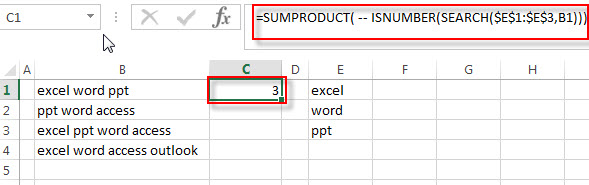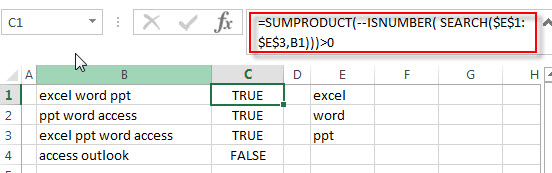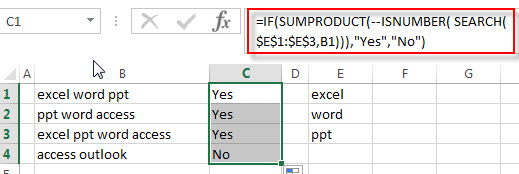# How to check if Cell contains one of many values from range

In the previous post, we talked that how to check if cell contains all of values from a list in excel, and this post will guide you how to test a cell if it contains one of several values in a range or a list in excel. How to check that at least one value in a list can be found in another range or a list.

## check if Cell contains one of many values from range

Assuming that you have a list of text strings in the range B1:B3 and you want to check each text string if it contains one of several values in a range E1:E3. If it contains any of text string in range E1:E3, then it should be return TRUE value, otherwise, it should return FALSE. You can use a combination of the SUMPRODUCT function, the ISNUMBER function and the SEARCH function to create a new excel formula as follows:

`=SUMPRODUCT(--ISNUMBER( SEARCH(\$E\$1:\$E\$3,B1)))>0`

Let’s see how this formula works:

=SEARCH(\$E\$1:\$E\$3,B1)

The SEACH function returns position of the first character of find_text in a text string. And this formula will search each value in the range E1:E3 inside within_text in Cell B1, then returns position of each text string in Cell B1, so it will return an array result like this:

`{1;7;12}`

The returned result goes into the ISNUMBER function as its argument.

=ISNUMBER(SEARCH(\$E\$1:\$E\$3,B1))

The ISNUMBER function will check if a cell contains a numeric value, and this formula will check each items of array result returned by the SEACH function, if the item is a numeric value, then return TRUE, otherwise, returns FALSE. So it will return another array result like this:

`{TRUE;TRUE;TRUE}`

=– ISNUMBER(SEARCH(E1:E3,B1))

The double-dash is known as a double unary operator, it can convert the TRUE values to 1 and FALSE values to 0. So this formula returns an array of numbers like this:

`{1,1,1}`

=SUMPRODUCT( — ISNUMBER(SEARCH(\$E\$1:\$E\$3,B1)))The SUMPRODUCT returns a total sum of the array result returned by the ISNUMBER function with double-dash operator. So it returns 3.

=SUMPRODUCT(–ISNUMBER( SEARCH(\$E\$1:\$E\$3,B1)))>0

If the result returned by the SUMPRODUCT is greater than the number of items in the range E1:E3, then we can know that at least one value in range E1:E3 can be found in Cell B1. So it returns TRUE, otherwise, this formula returns FALSE.Then you can drag the fill handle down to the cells that you want to apply this formula to check if a cell contains one of several values in another range E1:E3.

And if you want to return “yes” or “no” instead of “TRUE” or “FALSE”, then you can use the above SUMPRODUCT function to combine with the IF function to create a new formula as follows:

`=IF(SUMPRODUCT(--ISNUMBER( SEARCH(\$E\$1:\$E\$3,B1))),"Yes","No")`### Related Functions

• Excel SEARCH function
The Excel SEARCH function returns the number of the starting location of a substring in a text string.The syntax of the SEARCH function is as below:= SEARCH  (find_text, within_text,[start_num])…
• Excel SUMPRODUCT function
The Excel SUMPRODUCT function multiplies corresponding components in the given one or more arrays or ranges, and returns the sum of those products.The syntax of the SUMPRODUCT function is as below:= SUMPRODUCT (array1,[array2],…)…
• Excel ISNUMBER function
The Excel ISNUMBER function returns TRUE if the value in a cell is a numeric value, otherwise it will return FALSE.The syntax of the ISNUMBER function is as below:= ISNUMBER (value)…
• Excel COUNTA function
The Excel COUNTA function counts the number of cells that are not empty in a range. The syntax of the COUNTA function is as below:= COUNTA(value1, [value2],…)…
• Excel IF function
The Excel IF function perform a logical test to return one value if the condition is TRUE and return another value if the condition is FALSE.The syntax of the IF function is as below:= IF (condition, [true_value], [false_value])…